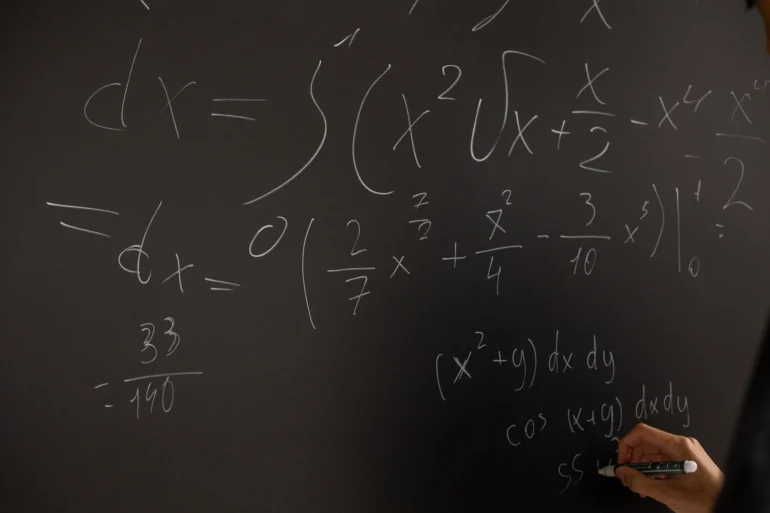# Understanding Back-Propagation in AI

Back-propagation is a critical component of artificial neural networks, which are used in many modern AI applications. This technique allows these networks to learn and improve their performance over time. In this article, we will explain the concept of back-propagation in simple terms, without using too much technical jargon.

Artificial neural networks are computer systems designed to learn and perform tasks in a similar way to the human brain. They consist of many interconnected processing nodes, called neurons, that work together to process information. Each neuron takes in one or more inputs and produces an output, which is then passed on to the next neuron in the network. The strength of the connection between neurons is represented by a weight value.

To train a neural network, we need to provide it with a large amount of data, which is used to adjust the weights between the neurons. The goal is to minimize the difference between the network’s output and the desired output for a given input.

Back-propagation is the technique used to adjust the weights in the neural network based on the error between the actual output and the desired output. The process involves propagating the error backwards through the network and adjusting the weights accordingly.

To understand this process, let’s take a simple example of a neural network that is trying to recognize handwritten digits. We can represent each digit as a 28×28 pixel image, with each pixel represented by a numerical value between 0 and 255. The neural network takes in this image as input and produces a probability distribution over the 10 possible digits (0-9).

Initially, the weights between the neurons are set randomly. When we provide an image of a digit as input, the network produces an output which is likely to be incorrect. We then compare this output to the desired output (the correct digit) and calculate the error.

The back-propagation algorithm involves propagating this error backwards through the network, layer by layer, and adjusting the weights based on the error at each layer. This process is repeated many times, using different examples from the training data, until the network’s performance improves.

The basic steps involved in back-propagation are as follows:

1. Feed the input data forward through the network, and calculate the output of each neuron.
2. Calculate the error between the actual output and the desired output.
3. Propagate the error backwards through the network, layer by layer, using the chain rule of calculus to calculate the contribution of each neuron to the error.
4. Use the calculated error to adjust the weights between the neurons, in order to reduce the error.
5. Repeat the process with a new example from the training data.

Over time, the weights between the neurons are adjusted in such a way that the network is able to produce more accurate outputs for a given input. This process of adjusting the weights based on the error is known as learning.

Back-propagation is a powerful technique for training neural networks, but it does have some limitations. One issue is that it can be slow, especially for large networks with many layers. Another issue is that it can sometimes get stuck in local minima, which are suboptimal solutions that the algorithm converges to.

Despite these limitations, back-propagation remains one of the most widely used techniques for training artificial neural networks. It has been applied successfully to many different applications, including speech recognition, image classification, and natural language processing.

In conclusion, back-propagation is a technique used to adjust the weights between neurons in an artificial neural network, based on the error between the actual output and the desired output. This allows the network to learn from training data and improve its performance over time. While back-propagation can be slow and prone to getting stuck in local minima, it remains a powerful technique for training neural networks.